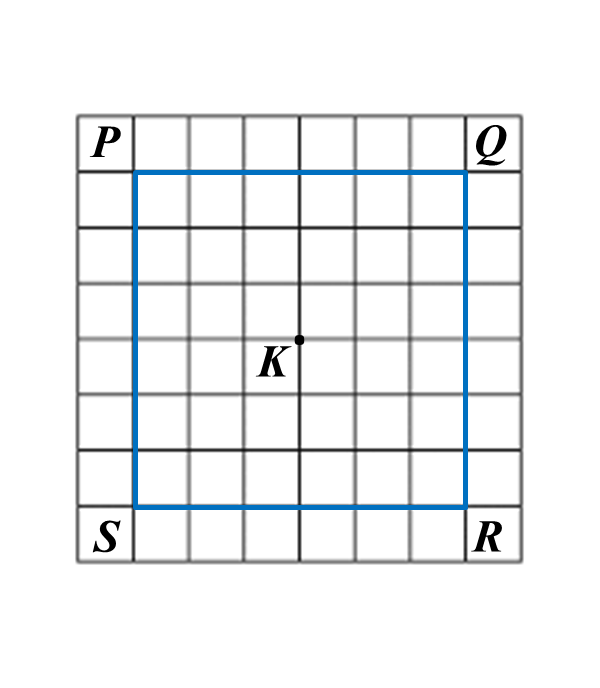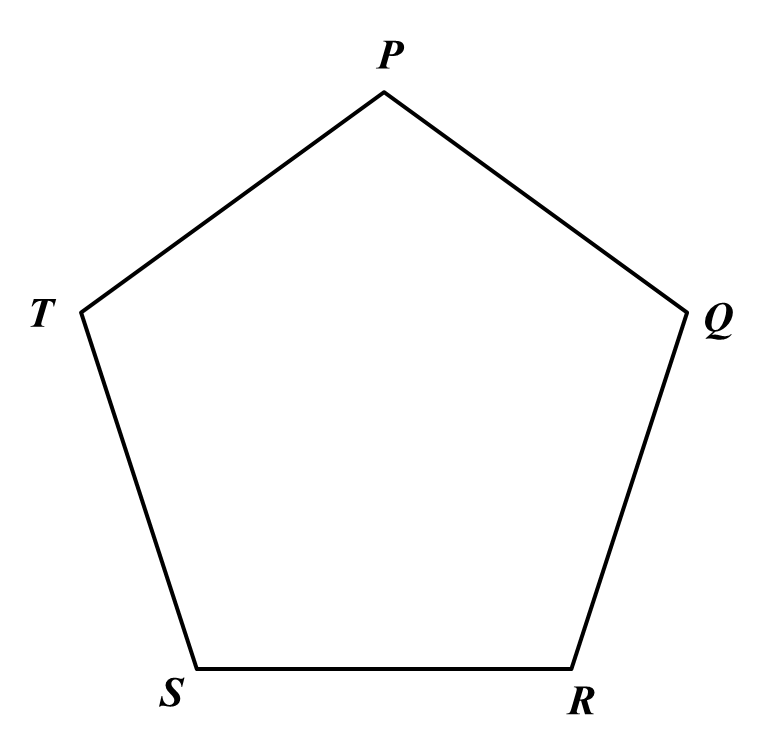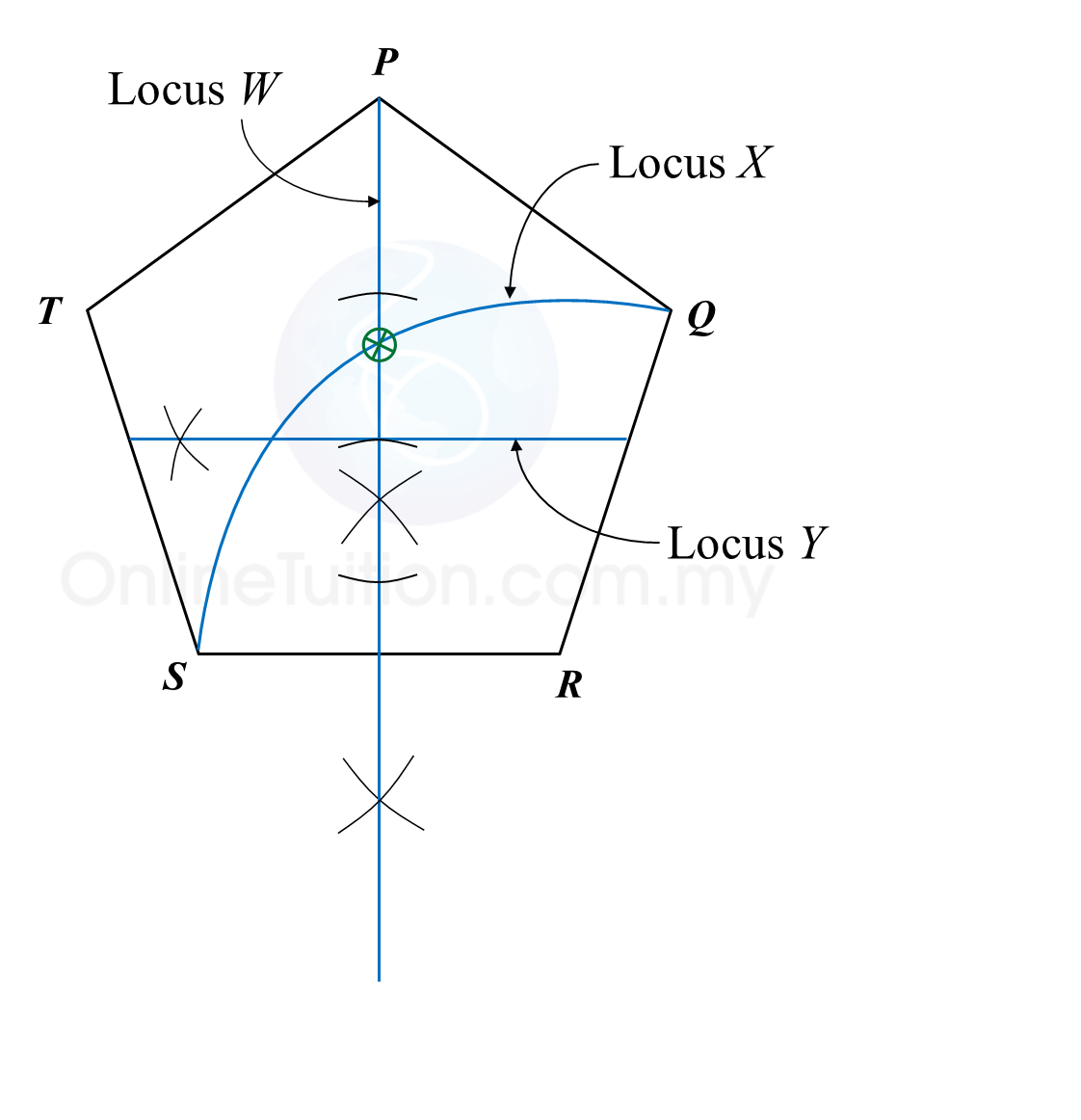# 8.2.1 Loci in Two Dimensions, PT3 Focus Practice

 
                    
                    
8.2.1 Loci in Two Dimensions, PT3 Focus Practice
                    

                    
Question 1:
                    
Diagram below in the answer space shows a square PQRS with sides of 6 units drawn on a grid of equal squares with sides of 1 unit. W, X and Y are three moving points inside the square.
 
 
                    
(a) W is the point which moves such that it is always equidistant from point P and point R.
                    
By using the letters in diagram, state the locus of W.
 
 
                    
(b) On the diagram, draw,
                    
(i) the locus of the point X which moves such that it is always equidistant from the straight lines PQ and PS,
                    
(ii) the locus of the point Y which moves such that its distance is constantly 2 units from point K.
 
 
                    
(c) Hence, mark with the symbol ⊗ the intersection of the locus of X and the locus of Y.
                    
  
                    

  Answer
:
                    
(b)(i),(ii)Solution:
                    
(a) QS
                     

 (b)(i),(ii)
                    
(c)Question 2:
                    
Diagram in the answer space below, shows a regular pentagon PQRST. W, X and Y are moving points which move in the pentagon.
                    
On the diagram,
 
 
                    
(a) draw the locus of the point W which moves such that it is always equidistant from point R and S.
 
 
                    
(b) draw the locus of the point X which moves such that XR = RS.
 
 
                    
(c) draw the locus of point Y which moves such that its distance is constantly 3 cm from the line SR.
 
 
                    
(d) hence, mark with the symbol ⊗ the intersection of the locus of W and the locus of X.
                    
  
                    

  Answer
:
                    
(a), (b), (c) and (d)Solution:
 (a), (b), (c) and (d)